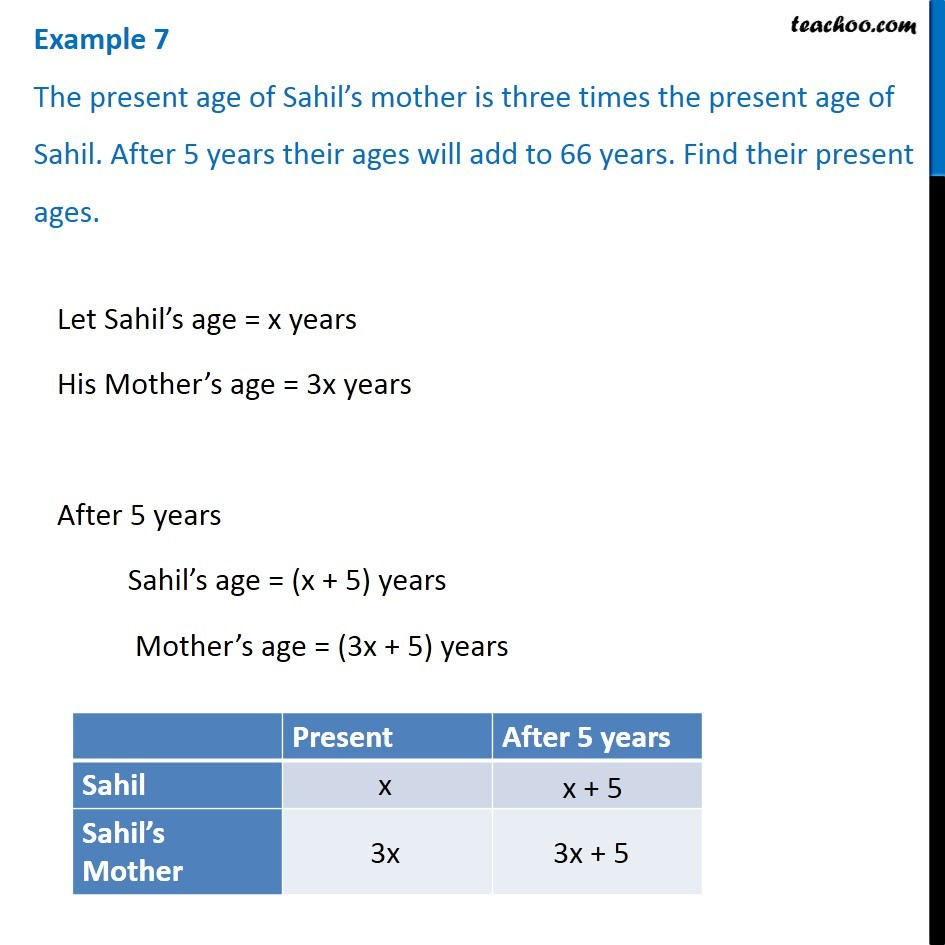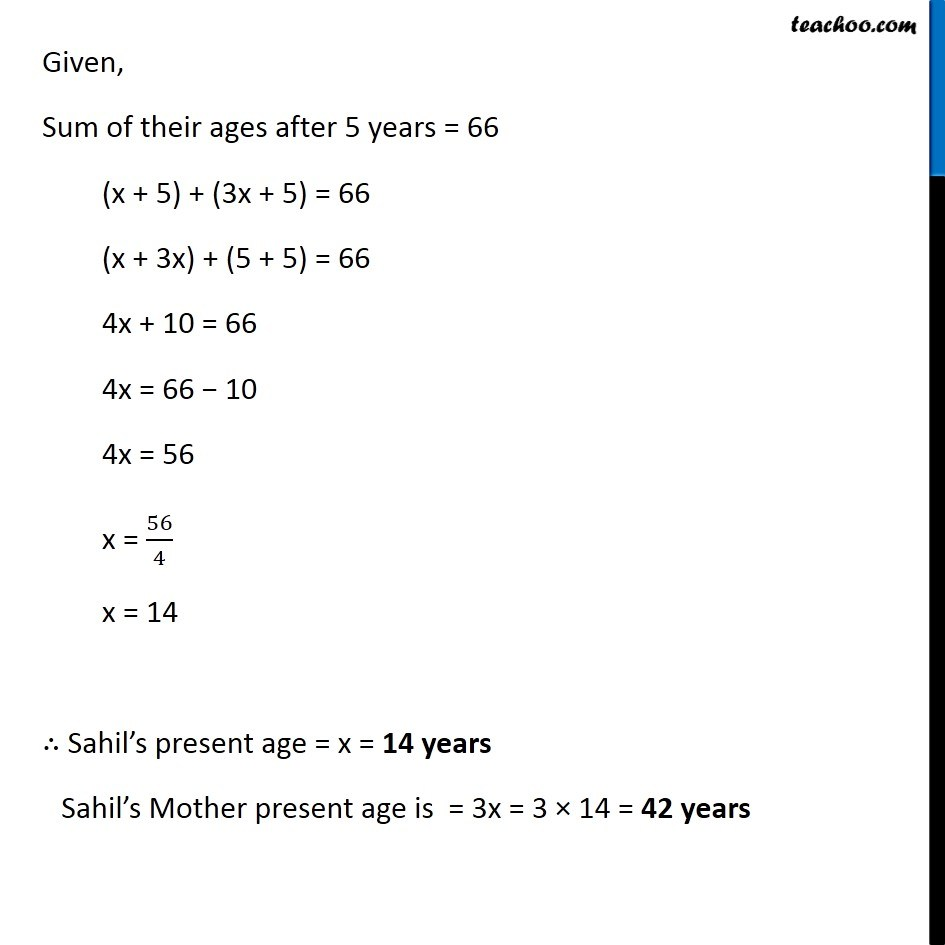Examples

Chapter 2 Class 8 Linear Equations in One Variable
Serial order wiseLearn in your speed, with individual attention - Teachoo Maths 1-on-1 Class

### Transcript

Question 7 The present age of Sahil’s mother is three times the present age of Sahil. After 5 years their ages will add to 66 years. Find their present ages.Let Sahil’s age = x years His Mother’s age = 3x years After 5 years Sahil’s age = (x + 5) years Mother’s age = (3x + 5) years Given, Sum of their ages after 5 years = 66 (x + 5) + (3x + 5) = 66 (x + 3x) + (5 + 5) = 66 4x + 10 = 66 4x = 66 − 10 4x = 56 x = 56/4 x = 14 ∴ Sahil’s present age = x = 14 years Sahil’s Mother present age is = 3x = 3 × 14 = 42 years